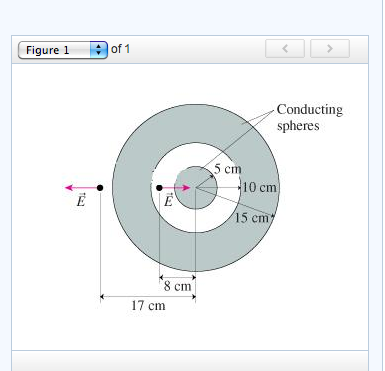# Problem: The figure (Figure 1) shows a solid metal sphere at the center of a hollow metal sphere. Assume that E = 1.3x104 N/C1) What is the total charge on the exterior of the inner sphere?2) What is the total charge on the inside surface of the hollow sphere?3) What is the total charge on the exterior surface of the hollow sphere?

###### FREE Expert Solution
92% (30 ratings)View Complete Written Solution
###### Problem Details

The figure (Figure 1) shows a solid metal sphere at the center of a hollow metal sphere. Assume that E = 1.3x104 N/C1) What is the total charge on the exterior of the inner sphere?

2) What is the total charge on the inside surface of the hollow sphere?

3) What is the total charge on the exterior surface of the hollow sphere?